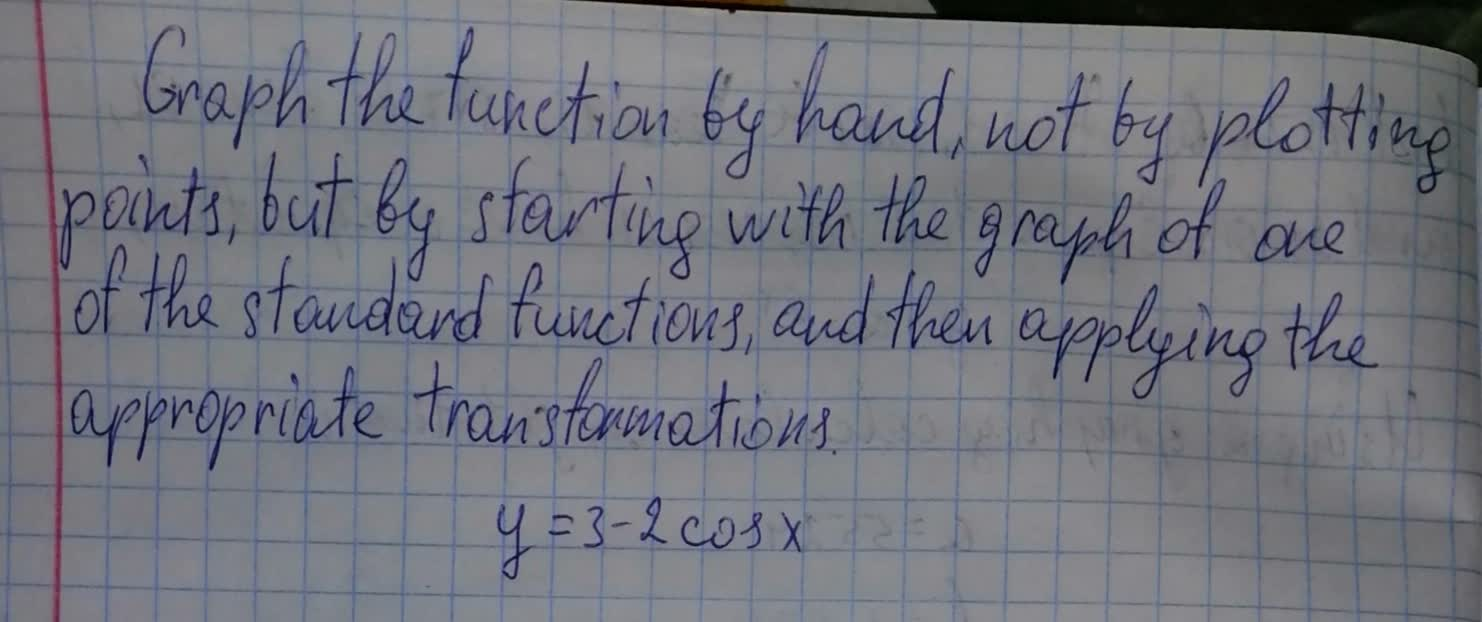# Solve problems related to transformations of functions with confidence, thanks to expert examples and equations.

Recent questions in Transformations of functionsbabeeb0oL 2021-09-12

## Find the limit and discuss the continuity of the function. $\underset{x,y}{lim}\to \left(2\pi ,4\right)\mathrm{sin}\frac{x}{y}$banganX 2021-08-16

## For each of the following functions f (x) and g(x), express g(x) in the form $a:f\left(x+b\right)+c$ for some values a,b and c, and hence describe a sequence of horizontal and vertical transformations which map $f\left(x\right)={x}^{2},g\left(x\right)=2{x}^{2}+4x$ $\left(ii\right)f\left(x\right)={x}^{2},g\left(x\right)=3{x}^{2}-24x+8$ $\left(b\right)\left(i\right)f\left(x\right)={x}^{2}+3,g\left(x\right)={x}^{2}-6x+8$ $\left(ii\right)f\left(x\right)={x}^{2}-2,g\left(x\right)=2+8x-4{x}^{2}$aflacatn 2021-08-15

## For $y={\mathrm{log}}_{3}\left(x+2\right)$ a. Use transformations of the graphs of $y={\mathrm{log}}_{2}x$ and $y={\mathrm{log}}_{3}x$ to graph the given functions. b. Write the domain and range in interval notation. c. Write an equation of the asymptote.hexacordoK 2021-08-14

## Graph the function by hand, not by plotting points, but by starting with the graph of one of the standard functions, and then applying the appropriate transformations. $y=2\sqrt{x+1}$avissidep 2021-08-14

## Begin by graphing $f\left(x\right)={2}^{x}$ Then use transformations of this graph to graph the given function. Be sure to graph and give equations of the asymptotes. Use the graphs to determine each functionsCoormaBak9 2021-08-14

## Sketch a graph of the function. Use transformations of functions whenever possible. $f\left(x\right)=-\frac{1}{{x}^{2}}$a2linetagadaW 2021-08-14

## For $y=2+{\mathrm{log}}_{3}x$. a) Use transformations of the graphs of o graph the given functions. b) Write the domain and range in interval notation. c) Write an equation of the asymptote.Reggie 2021-08-13

## In your earlier work in algebra, you learned how to recognize linear, exponential, and quadratic functions by the form of their symbolic rules. Geometric transformations also can be recognized by their symbolic rules. What transformation is defined by each of the following coordinate rules?bobbie71G 2021-08-12

## Describe the transformations that were applied to $y={x}^{4}$ to get each of the following functions. $d\right)y=\frac{8}{11}{\left(-x\right)}^{4}-1$shadsiei 2021-08-11

## Graph the functions $y={5}^{x},y=-{5}^{x},y={5}^{x}+2,y={5}^{x}-2$, and $y={10}^{x}$ on the same screen. Compare $y=-{5}^{x},y={5}^{-x}$ to the parent graph $y={5}^{x}$. Describe the transformations of the functions.Phoebe 2021-08-08

## Begin by graphing the standard quadratic functions. $f\left(x\right)={x}^{2}$. Then use transformations of this graph to the given function. $r\left(x\right)=-{\left(x+1\right)}^{2}$Clifland 2021-08-08

## Graph the function by hand, not by plotting points, but by starting with the graph of one of the standard functions, and then applying the appropriate transformations. $y={\left(x-3\right)}^{2}$vazelinahS 2021-07-30

## Describe the transformations that were applied to $y={x}^{2}$ to obtain each of the following functions. $e\right)y=40{\left(-7\left(x-10\right)\right)}^{2}+9$Ava-May Nelson 2021-07-13

## Graph the function by hand, not by plotting points, but by starting with the graph of one of the standard functions, and then applying the appropriate transformations. $y=3-2\mathrm{cos}x$naivlingr 2021-07-04

## g is related to one of the parent functions described in Section 1.6. Describe the sequence of transformations from f to g. $g\left(x\right)=\sqrt{\frac{1}{4}}x$glasskerfu 2021-06-29

## g is related to one of the parent functions described in Section 1.6. Describe the sequence of transformations from f to g. $g\left(x\right)=3-||x||$floymdiT 2021-06-26

## The process by which we determine limits of rational functions applies equally well to ratios containing noninteger or negative powers of x: Divide numerator and denominator by the highest power of x in the denominator and proceed from there. Find the limits. $\underset{x\to \mathrm{\infty }}{lim}\frac{x-3}{\sqrt{4{x}^{2}+25}}$Jason Farmer 2021-06-21

## g is related to one of the parent functions Describe the sequence of transformations from f to g. $g\left(x\right)=\frac{1}{2}|x-2|-3$Reeves 2021-06-20
## Determine the domains of the given rational functions. $\frac{{x}^{2}-9}{{x}^{3}-x}$sanuluy 2021-06-18